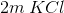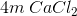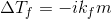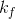# MCAT Physical : Freezing Point

## Example Questions

2 Next →

### Example Question #57 : Solution Chemistry

Colligative properties are properties of compounds that are altered by the amount of substance present. There are four main colligative properties: boiling point, freezing point, vapor pressure, and osmotic pressure. The change in each of these properties can be calculated using the amount of molecules/ions present in solution and the concentration or partial pressure of the compound. The boiling point is defined as the temperature at which the vapor pressure equals the atmospheric pressure. The freezing point is the temperature at which a liquid is converted to a solid. Vapor pressure is the pressure produced by the vapor above a solution. Osmotic pressure is the pressure required to prevent flow of water into a solution (across a membrane).

Consider the following two solutions:

Solution A:Solution B:Which of the following is true regarding these two solutions?

Solution A has 2 times higher freezing point

Solution B has 2 times higher freezing point

Solution A has 3 times higher freezing point

Solution B has 3 times higher freezing point

Solution A has 3 times higher freezing point

Explanation:

The freezing point depression can be calculated by the following equation.whereis change in boiling point,is the freezing point depression constant,is the number of ions, andis the molality. Solution B will produce three ions whereas solution A will produce two ions in solution; therefore, if the concentration were the same, solution B will have a lower freezing point by a factor of 1.5. The concentration, however, is different. The concentration of solution B is twice as much as solution A; therefore, the freezing point of solution B will be lower by a factor of 3 (1.5 from ions and 2 from concentration); therefore, solution A will have 3 times higher freezing point.

2 Next →

### All MCAT Physical Resources